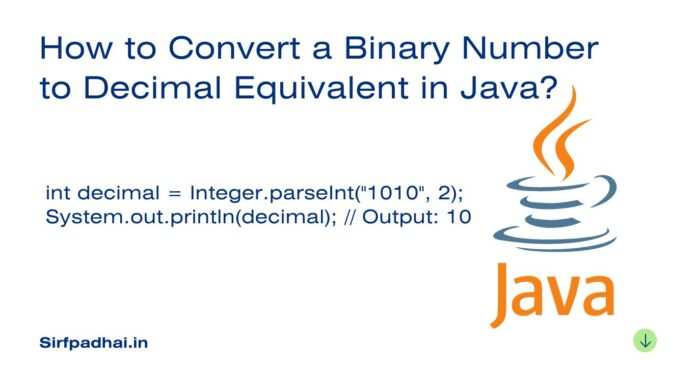# How to Convert a Binary Number to Decimal Equivalent in Java?## How to Convert a Binary Number to Decimal Equivalent in Java?

There are two ways to convert a binary number to a decimal equivalent in Java.

## 1. Using the `Integer.parseInt()` method

The `Integer.parseInt()` the method can be used to convert a binary number to a decimal equivalent. The syntax for using this method is as follows:

``int decimal = Integer.parseInt(binaryString, 2);``

where `binaryString` is the binary number that you want to convert and `2` is the base of the binary number.

For example, the following code will convert the binary number `1010` to decimal equivalent:

``````int decimal = Integer.parseInt("1010", 2);
System.out.println(decimal); // Output: 10``````

## 2. Using a custom method

You can also create a custom method to convert a binary number to a decimal equivalent. The following is a simple example of a custom method that can be used to do this:

```public static int getDecimal(int binary) {
int decimal = 0;
int n = 0;
while (true) {
if (binary == 0) {
break;
} else {
int temp = binary % 10;
decimal += temp * Math.pow(2, n);
binary /= 10;
n++;
}
}
return decimal;
}
```

This method works by first converting the binary number to a string. Then, it loops through the string, one digit at a time. For each digit, it multiplies the digit by the power of 2, where the power is equal to the index of the digit in the string. Finally, it adds all of the products together to get the decimal equivalent of the binary number.

For example, the following code will use the `getDecimal()` method to convert the binary number `1010` to decimal equivalent:

``````int decimal = getDecimal(1010);
System.out.println(decimal); // Output: 10
``````